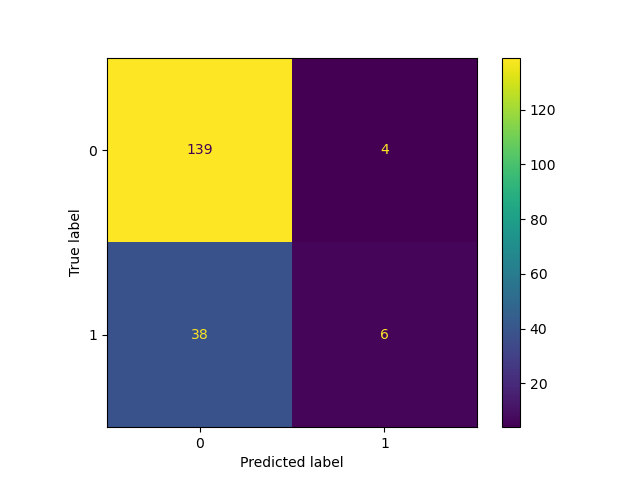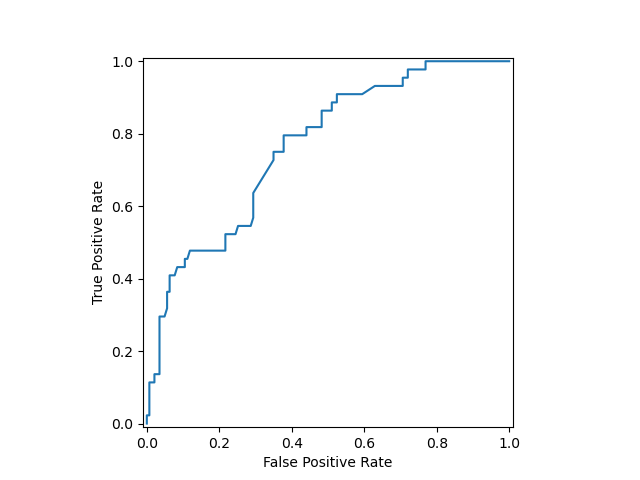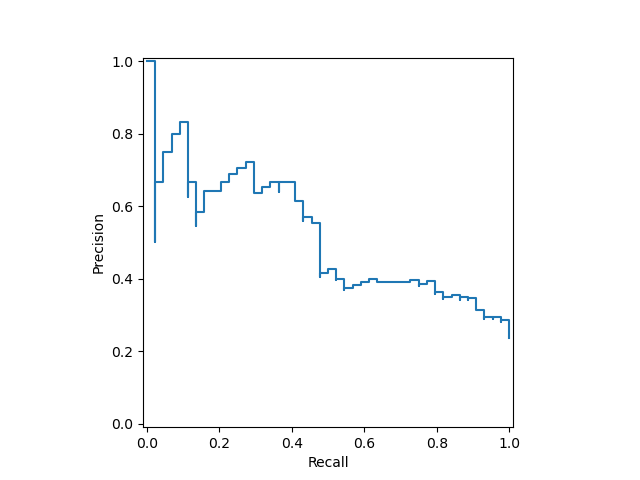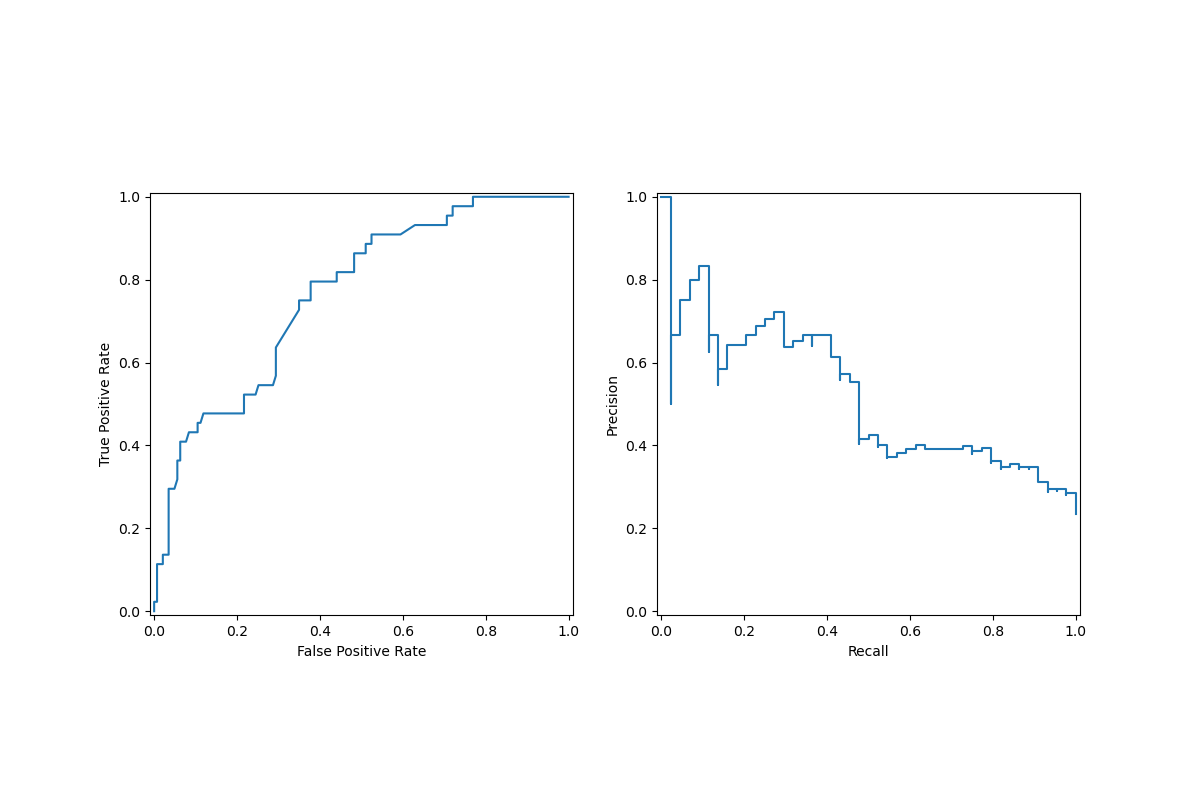# Visualizations with Display Objects¶

In this example, we will construct display objects, ConfusionMatrixDisplay, RocCurveDisplay, and PrecisionRecallDisplay directly from their respective metrics. This is an alternative to using their corresponding plot functions when a model’s predictions are already computed or expensive to compute. Note that this is advanced usage, and in general we recommend using their respective plot functions.

## Load Data and train model¶

For this example, we load a blood transfusion service center data set from OpenML <https://www.openml.org/d/1464>. This is a binary classification problem where the target is whether an individual donated blood. Then the data is split into a train and test dataset and a logistic regression is fitted with the train dataset.

from sklearn.datasets import fetch_openml
from sklearn.linear_model import LogisticRegression
from sklearn.model_selection import train_test_split
from sklearn.pipeline import make_pipeline
from sklearn.preprocessing import StandardScaler

X, y = fetch_openml(data_id=1464, return_X_y=True, parser="pandas")
X_train, X_test, y_train, y_test = train_test_split(X, y, stratify=y)

clf = make_pipeline(StandardScaler(), LogisticRegression(random_state=0))
clf.fit(X_train, y_train)

Pipeline(steps=[('standardscaler', StandardScaler()),
('logisticregression', LogisticRegression(random_state=0))])
In a Jupyter environment, please rerun this cell to show the HTML representation or trust the notebook.

### Create ConfusionMatrixDisplay¶

With the fitted model, we compute the predictions of the model on the test dataset. These predictions are used to compute the confusion matrix which is plotted with the ConfusionMatrixDisplay

from sklearn.metrics import ConfusionMatrixDisplay, confusion_matrix

y_pred = clf.predict(X_test)
cm = confusion_matrix(y_test, y_pred)

cm_display = ConfusionMatrixDisplay(cm).plot()### Create RocCurveDisplay¶

The roc curve requires either the probabilities or the non-thresholded decision values from the estimator. Since the logistic regression provides a decision function, we will use it to plot the roc curve:

from sklearn.metrics import RocCurveDisplay, roc_curve

y_score = clf.decision_function(X_test)

fpr, tpr, _ = roc_curve(y_test, y_score, pos_label=clf.classes_)
roc_display = RocCurveDisplay(fpr=fpr, tpr=tpr).plot()### Create PrecisionRecallDisplay¶

Similarly, the precision recall curve can be plotted using y_score from the prevision sections.

from sklearn.metrics import PrecisionRecallDisplay, precision_recall_curve

prec, recall, _ = precision_recall_curve(y_test, y_score, pos_label=clf.classes_)
pr_display = PrecisionRecallDisplay(precision=prec, recall=recall).plot()### Combining the display objects into a single plot¶

The display objects store the computed values that were passed as arguments. This allows for the visualizations to be easliy combined using matplotlib’s API. In the following example, we place the displays next to each other in a row.

import matplotlib.pyplot as plt

fig, (ax1, ax2) = plt.subplots(1, 2, figsize=(12, 8))

roc_display.plot(ax=ax1)
pr_display.plot(ax=ax2)
plt.show()Total running time of the script: (0 minutes 0.338 seconds)

Gallery generated by Sphinx-Gallery